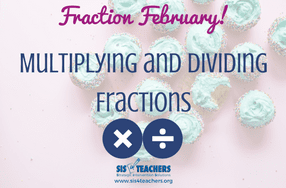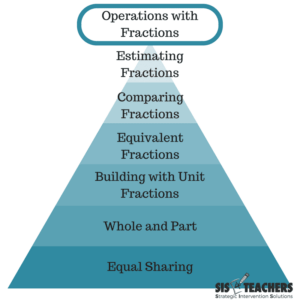Select Page

# Fraction February: Multiplication and Division

Mar 1, 2018

If we think about how we learned multiplication and division of fractions, it’s actually quite comical.Many of us were taught to just multiply across. So, when kids look at whole number multiplication and they transition into fractions, they really don’t make a whole lot of connections besides just multiplying the numerators and the denominators and ending up with an answer. They really don’t have any idea what is happening conceptually with fractions. Some kids don’t even make the connection that, when multiplying fractions, the number gets smaller, where when we multiply whole numbers, the number actually gets larger.

### Multiplying

Start by presenting a multiplication problem, 6 x 4 for example, and ask the students what that actually means. Most of the students will tell you, “That it means multiplication!” Yes, but what does that mean? “It means you’re going to times!” Yes, but what does the statement mean? We have to get kids to understand what multiplication is; that when says 6 x 4, what it’s really saying is 6 groups of 4.

If kids understand that multiplication doesn’t mean just “timesing” or multiplying but that it actually means a certain amount of groups of something – when we go into looking at the concept of multiplication in fractions, we can connect that understanding.

For example, if I had ½ x ⅓, what does that mean? It means ½ of ⅓. What does that look like with pattern blocks? In this example, we’ll go back to the hexagon as our whole, which would make the trapezoid the half, rhombus thirds, and the triangles sixths. The idea is that kids can have a visual for what we’re talking about when we say ½ x ⅓. If the hexagon is the whole, the piece I should be looking at is the blue rhombus because that represents ⅓. Hold that rhombus in your hand and ask yourself the question again – what is ½ of ⅓? As you look at it, you can easily see that the answer will be the green triangle, which represents ⅙ of my whole. A child building a model with the pattern blocks would show me their blue rhombus, knowing that the hexagon is the whole, and then have a green triangle on top of it to be able to point to what one half of one third is.

We might also look at the commutative property – does the model look different when we use the commutative property within fractions? Now have ⅓ x ½. Is the model the same? Is it the blue rhombus? Or is it asking something different?

I might say ⅓ OF ½…so the shape I need in my hand is the red trapezoid. I look at it again and ask myself, “what is ⅓ x ½?” As I hold the shape I can see how it gets broken into three pieces. I know that one of them will be the green triangles. So, the model for this one would have the hexagon as the whole, the trapezoid with a green triangle on top to model what ⅓ x ½ would be.

It’s really important that kids understand what they’re doing, and that they’re not just doing 1 times 1 or 3 times 2 because they aren’t even whole numbers. Students need to understand the concept of what’s happening in multiplication before they can even attempt to understand fractions.

### Dividing

Division is the other area of fractions that is very confusing. One of the resources we talked about early in our Fraction February was Beyond Pizzas and Pies, but we also love Beyond Invert and Multiply. Kids just know to “not ask why, just invert and multiply.”  But we need to ask why. Why are you doing that? Why are you getting a whole number when you divide fractions? That doesn’t make much sense! Why am I flipping one of the numbers and making it a whole number?

This video is a great explanation of how to show division of fractions with pattern blocks, getting kids to really understand what it means.

For division, I would pose the question the same way we did when we started multiplication. Put the problem on the board: 36 / 4. Ask what does that statement mean? Kids will say, “it means divide by.” But it is really asking, “how many groups of 4 are in 36?” So, as we transition from whole numbers to fractions, we want kids to ask the same questions – how many groups are in that number?

Now, if I put up a fraction problem: ½ / 1/6 .  Ask the students what that really means. It means how many sixths are in ½? If I’m wondering how many sixths are in ½, I’m again going to start thinking about that hexagon as the whole and my half will be the red trapezoid. So I put that red trapezoid down and ask myself again: “How many sixths are in my half?” I know my sixth is the green triangle, so, as I put the sixths on top of the half, I can see how sixths are in one half.

This is a simplistic start to division, but in the video, they go into much more depth when looking at a fraction that might have a remainder. If I was looking at ⅚ and was trying to figure out how many thirds were in there, ⅚ tells me that at least 2 thirds will go in there, but I will actually have a little bit left over, and my answer is 2 ½ .

Some kids get confused when you do division with pattern blocks, but it just requires repetition and getting them to think outside the box and think conceptually about what the problem is actually asking. This lends itself to discovery that we want kids to make. We’re not doing any kind of flipping or butterfly method or an of those types of procedures with concepts kids don’t understand, but we want kids to look at the problem and think about the conceptual experience. Why do they get a whole number when they’re dividing? Why they are trying to see how many of that one number could  go into the other number?

For many children, if you skip this conceptual piece, it’s really doing them a disservice. When you look at the the 8 Standards of Mathematical Practice and what’s being asked of the kids in this 21st century, it is not just a regurgitation of information. In Michigan, we do MSTAT testing, and recently, we were analyzing the type of questions the test was asking. If kids have just memorized a list of procedures with concepts they don’t know or understand, quite frankly they’re not going to do well on 75% of the state test because the test is asking asking for the application of what the students understand.

One of the questions on the test was 4 x ¾. The test didn’t want the answer. It wanted the student to pick which picture modeled the problem. If kids have not learned that “4 x ¾” means 4 groups of ¾, they won’t be able to pick the correct picture. Even if they know the answer, it’s not going to matter because, conceptually, they actually have to understand fractions to determine the correct model.

### Beyond Fraction February

Of course, there is a lot more fraction work we could do that we can’t fit into this blog series! We could talk about using number lines, tape diagrams or any other manipulative. When choosing a manipulative, make sure to select one that kids can visualize and recall in their head. Kids are so familiar with pattern blocks because they’ve used them since Kindergarten, so they are a friendly tool that students can start to experiment with.As you work with students to build conceptual understanding, remember our pyramid of steps that kids must follow in order to be ready for the next thing in fractions. We don’t want to rush them into it! We want to make sure that we follow the progression of concepts, carefully solidifying their understanding on one level before building on more advanced ideas.